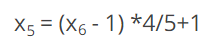top of page
Search
• Lara Winkless

# There and Back Again: Can I Change a 6-Point Scale to a 5-Point Scale?

Updated: May 3

When you run a survey for multiple years, you get to see the big picture: What changed, and how effective different approaches were. Although the benefits are worth it, long-term projects do involve some challenges. A certain question may no longer apply, or a new hot topic arose requiring a new question, or you’d like to change the survey methodology. One situation we’ve encountered is when the survey needs a new Likert scale such as, for example, changing a 6 point scale to a 5 point scale, while preserving the ability to compare results.

## Likert Scales

Likert scales ask participants to rate how much they agree or disagree with a statement. If you’ve ever taken a survey, you’ve probably encountered a Likert scale: that’s just how popular they are. Likert scales allow us to quantify opinions we usually think of as subjective and unquantifiable. Of course, they do have some weaknesses, such as positivity bias, which is the tendency for participants to choose a more positive option than how they actually feel.

Likert scales range from two to ten points, with each value having its own advantages and disadvantages. If your organization has decided to change a survey’s scale, they’ve probably spent some time weighing each one and discussing the best fit. Thanks to mathematics, whichever scale you choose you don’t have to sacrifice the option of being able to trend the data against past results. Although it's a technical process, we can “translate” results from one scale to another through linear interpolation.## Linear Interpolation

Linear interpolation is the process of finding the value of one variable on a line within the range of an already established dataset using the value of another variable. The existing relationship between the variables allows us to input one number and receive the output of a different number that preserves the mathematical relationship between them. In our example, the first variable is the 6-point scale, and the other variable (the value produced by the function) is the 5-point scale.

## How to Change a 6-Point Scale to a 5-Point Scale: The Technical Details

The specific formula of the function is:Using this formula, we can transform the current 6-point scale ranging from 1 to 6 to a 5-point scale ranging from 1 to 5.

To adjust the scale from 6 points to 5 points, we subtract 1 from the 6 point scale to transform it into a scale of 0 to 5. If we skipped this step, we would find ourselves starting at a value that is lower than 1 in the new scale, which wouldn’t make any sense, since 1 corresponds to the lowest value. From there, we convert the 0 to 5-point scale into a 0 to 4-point scale by multiplying all values by a factor of 4/5. Once this is done, we need to convert the new 0 to 4 scale into a 1 to 5 scale, shifting the scale by adding 1.

In this case, a ‘6’ from the original scale would look like this: (6-1)*4/5 +1 = 5;

A ‘3’ from the original scale would look like: (3-1)*4/5+1 = 2.6; and

A ‘1’ form the original scale would look like: (1-1)*4/5 +1 = 1

If we wanted to reverse the process and transform a 5 point scale into a 6 point, there is not much that would need to be changed. We would still subtract 1 from the 5 point scale to transform it into a scale of 0 to 4, then convert this scale into a 0 to 5-point scale by multiplying all values by a factor of 5/4. The fraction will always be structured as such: the numerator will be the new scale’s length minus 1, and the denominator will be the old scale’s length minus 1. Finally, like the original process, we convert the new 0 to 5 scale into a 1 to 6 scale, shifting the scale by adding 1.

In this case, a ‘5’ from the original scale would look like this: (5-1)*5/4 +1 = 6;

A ‘3’ from the original scale would look like: (3-1)*5/4+1 = 3.5; and

A ‘1’ form the original scale would look like: (1-1)*5/4 +1 = 1

## A Weighted Approach

Sometimes, part of the reason why the survey needs a new scale is because participants weren’t selecting certain responses, indicating that some options were unnecessary. If this was the case, you may want to consider a less literal approach when translating old survey results to the new scale. A weighted approach involves adjusting the formula depending on how significant the responses to that question were to the overall data. While a weighted approach features added complexity and subjectivity, in cases where it’s warranted it will likely make the results easier to interpret.

Whichever method you choose, ultimately you'll be able to easily compare your results from those from years past, providing a rich dataset to support change and growth.

If you found this tutorial useful, you might enjoy our newsletter. It’s a monthly email that keeps you up to date on what we’re up to and articles on topics we find interesting.

If you're thinking of implementing a survey for your organization or already run surveys and are encountering situations like this, sign up for a free, 30-minute consultation to see what Kai Analytics can do for you.